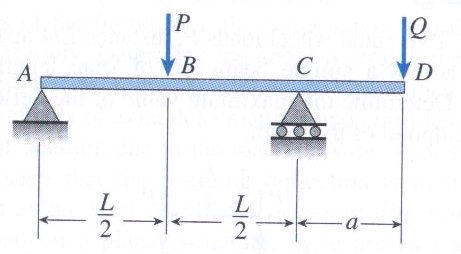Feb 26, 2016

Feb 26, 2016

Feb 26, 2016

# Determination of Deflection in Over Hanging Beams

## Apparatus:

Model of beam, Weights, Deflection Gauge, Weight Hangers.

### Objective:

The purpose of this experiment is to record the deflection in beam experimentally and then compare it with theoretical value.

### Deflection:

Deflection is a term which is defined as the distance moved by a point on the axis of beam before and after application of force

### Determination bar:

Those bars in which unknown reactions can be found using available egs of equilibrium are called determination.## Procedure of Experiment:

1. Take the beam model and place it on the table. it should be kept horizontally and firmly.

2. Determine the length of the beam and also dimension of cross section.

3. If the model is an over hanging bema then also determine the length of over hanging portion.

4. Set the deflection gauge at a point where deflection is to be measured.

5. Make the reading of the deflection gauge dial indicator to zero before applying the load on bar.

6. Now apply the load with the help of load hangers and record the loaded weights location from left side of the beam.

7. Now record the deflection 1st of all at the smaller dial of the gauge. It should be read as it shows the number of rotations.

8. One complete rotation is equal to 1mm deflection